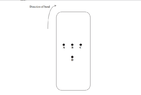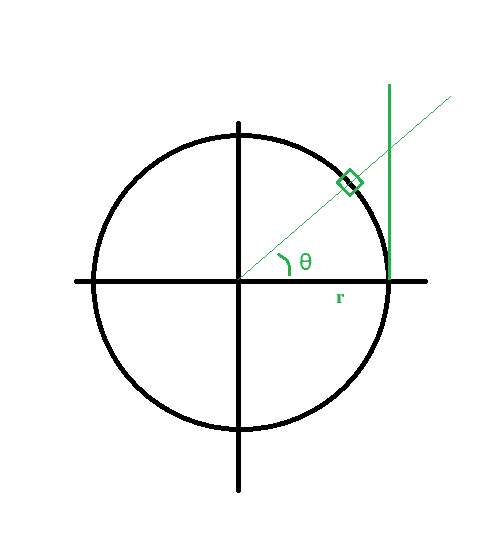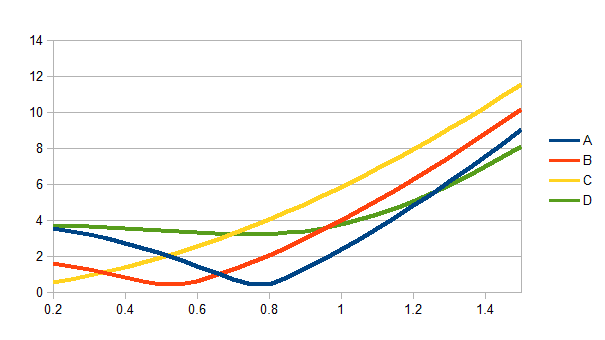# Statics & Resolving Forces

• shk

#### shk

Homework Statement
A person is standing at point C in a train carriage travelling round a sharp bend to the right. The person jumps up. Nearest which marked point is the person most likely to land?
Relevant Equations
F=ma
I need help with the following question please.
I understand that the person carries on in straight line while is jumping. This is because there is no horizontal force acting on him so based on Newton's first law of motion he continues with the previous speed.
I know he should land on A or B but I'm not sure why B cannot be the answer.

here is the question:

A person is standing at point C in a train carriage traveling round a sharp bend to the right. The person jumps up. Nearest which marked point is the person most likely to land?

I have attached the picture for you to see.

#### Attachments

•Physics. bus .png
4 KB · Views: 66

Why?

Homework Statement:: A person is standing at point C in a train carriage traveling round a sharp bend to the right. The person jumps up. Nearest which marked point is the person most likely to land?
.
I understand that the person carries on in straight line while is jumping. This is because there is no horizontal force acting on him so based on Newton's first law of motion he continues with the previous speed.
The question says ‘Nearest which marked point...’. So there is no reason to believe the person should land exactly on any of the marked points.

The question says ‘most likely to land’ which implies different answers may be feasible but with different probabilities. That’s very unsatisfactory in my view.

It is not clear what ‘sharp bend’ implies. Does this mean the bend is completed while the person is still in the air?

Note you wrote “he continues with the previous speed“.
This should be “he continues with the previous velocity”.
EDIT: I should have said: "he continues with the horizontal component of velocity unchanged.

I can’t see any way to reach a sensible answer. But that could just be me. I’d be interested to read what others say.

Edit: Note, your title "Statics & Resolving Forces" is completely inappropriate!

Last edited:
•Delta2 and shk
The answer as I see it is point C. I don't see a force acting on an "up" jump. Not a sideways jump, but an up jump. You will land in the same spot depending on the frame of reference.

If the question is actually asking where will you land depending on the ground under the train then I would say D. But that is not with in the frame of reference of the jumper.

The answer as I see it is point C. I don't see a force acting on an "up" jump. Not a sideways jump, but an up jump. You will land in the same spot depending on the frame of reference.
I'm not sure I'm following your appeal to reference frames here...

The rotation makes it non-intertial. There are fictitious forces that come into play. If you jump and your reference frame changes directions while you're in the air, why would you keep tracking with that frame?

While the train is turning, you feel that centrifugal force. If you've jumped into the air, there's no centripetal force holding you to the turn.

The answer as I see it is point C. I don't see a force acting on an "up" jump. Not a sideways jump, but an up jump. You will land in the same spot depending on the frame of reference.

If the question is actually asking where will you land depending on the ground under the train then I would say D. But that is not with in the frame of reference of the jumper.
If you are external observer on the ground, you will see this:

1. From Newton's 1st law, the person's horizontal velocity component while in the air will be constant; it is the same as at the moment he jumped up, i.e equal to the carriage's initial horizontal velocity. So the person will behave as a simple projectile, heading in the +y direction.

2. While the person is in the air, the centre of the carriage will have moved in the +y and +x directions. And the carriage's axis will have rotated clockwise 90º (assuming that's what is mean by 'travelling round a sharp bend to the right').

So, as far as I can see, the person cannot possibly land on point C.

•shk
Why?
I have explained that my answer is either A or B. I know the answer is A but not sure why .

Why?
I have explained that my answer is either A or B but not sure which one. I also know that the answer must be A .

If you are external observer on the ground, you will see this:

1. From Newton's 1st law, the person's horizontal velocity component while in the air will be constant; it is the same as at the moment he jumped up, i.e equal to the carriage's initial horizontal velocity. So the person will behave as a simple projectile, heading in the +y direction.

2. While the person is in the air, the centre of the carriage will have moved in the +y and +x directions. And the carriage's axis will have rotated clockwise 90º (assuming that's what is mean by 'travelling round a sharp bend to the right').

So, as far as I can see, the person cannot possibly land on point C.
Hi . Thank you. I know the answer is A but I cannot explain why .

I would draw this out, a bit exaggerated. Considering a sharp turn, I think that would imply a longer travel distance (i.e. increased speed) for both points A and B...

Are you sure D is out?

•shk
I've changed my thinking from Post #6. I think C ia a possible answer.

For example, suppose the person jumps up for 1 microsecond and the turn takes 10 seconds. Then the nearest landing point will be C because the person has not had sufficient time to move signficantly relative to the carriage.

This is an old GCE A level Edexcel question (Edexcel is a UK Examination Board). I know the official answer is 'A' but the question is poorly written and, in my opinion, can't be answered with the limited information supplied.

I was a 'marker' for Edexcel (and AQA) many years ago, so I know these sorts of 'bad' questions occasionally get through the system.

try drawing it like this. (left turn instead of right...)The distance traveled along the vertical green line should be the same length as a given arc segment, if the speed is constant.

•shk
If you are external observer on the ground, you will see this:

1. From Newton's 1st law, the person's horizontal velocity component while in the air will be constant; it is the same as at the moment he jumped up, i.e equal to the carriage's initial horizontal velocity. So the person will behave as a simple projectile, heading in the +y direction.

2. While the person is in the air, the centre of the carriage will have moved in the +y and +x directions. And the carriage's axis will have rotated clockwise 90º (assuming that's what is mean by 'travelling round a sharp bend to the right').

So, as far as I can see, the person cannot possibly land on point C.
A poorly written question will cause more confusion. If the jump is slight then C is the answer. If the turn and the centrifugal force is slight(what is the definition of sharp) then the answer is still C. However, if the speed of the train is fast and the turn truly sharp then depending on those factors(forces) you may land on any of them.

The poster stated they knew the answer was A, but how? What forces was provided with the question to arrive at that fact?

A poorly written question will cause more confusion. If the jump is slight then C is the answer. If the turn and the centrifugal force is slight(what is the definition of sharp) then the answer is still C. However, if the speed of the train is fast and the turn truly sharp then depending on those factors(forces) you may land on any of them.

The poster stated they knew the answer was A, but how? What forces was provided with the question to arrive at that fact?
It is not about forces, other than that the jumper continues with the same horizontal velocity because there is no horizontal force on her.
We can assume that the speed is also constant for the centre of the carriage. Since it is a train compartment, not a coach, the centre of curvature (once both bogeys are in the turn) will be on the left-to-right midline.
It is reasonable to assume a substantial turn, at least 30 degrees.

I took the centre of the turn as the origin, at distance r to the right of the centre of the carriage. Based on the diagram, I set the initial coordinates of A, B, C, D as (-r-2,1), (-r,1), (-r+2,1), (-r,-2).
With a turn through ##\theta##, the final position of the person is ##(-r+2,1+r\theta)##.
With r=10 I found:
Theta=pi/6, B is nearest (very close)
Theta=pi/4, A is nearest (very close)
Theta=pi/3, A is nearest
Theta=pi/2, D is nearest
Seems like this is a BAAD question.

Edit: to confirm @Steve4Physics' point in post ##11, C is nearest for theta less than about 19 degrees.

Last edited:
•SammyS
We did this a while back (can't find it, though).
What's the thread Title have to do with anything ?

We did this a while back (can't find it, though).
What's the thread Title have to do with anything ?

With the same settings as in post #14, here are the distances from the landing point to the labelled points (y) as a function of theta (x, radians):Last edited:
•hmmm27 and shk
The question says ‘Nearest which marked point...’. So there is no reason to believe the person should land exactly on any of the marked points.

The question says ‘most likely to land’ which implies different answers may be feasible but with different probabilities. That’s very unsatisfactory in my view.

It is not clear what ‘sharp bend’ implies. Does this mean the bend is completed while the person is still in the air?

Note you wrote “he continues with the previous speed“.
This should be “he continues with the previous velocity”.
EDIT: I should have said: "he continues with the horizontal component of velocity unchanged.

I can’t see any way to reach a sensible answer. But that could just be me. I’d be interested to read what others say.

Edit: Note, your title "Statics & Resolving Forces" is completely inappropriate!
I put the title of the exam paper thinking it was the right one.
I found the question in an exam paper from savemyexams website. the answer is A. There was no explanation since this was a multiple choice question.
In my opinion , the answer depends on how fast the bus was going and how sharp the bend is. But if the answer is A, there must be an explanation for it.
Thank you for the reply. :)

I would draw this out, a bit exaggerated. Considering a sharp turn, I think that would imply a longer travel distance (i.e. increased speed) for both points A and B...

Are you sure D is out?
Yes I am sure. In fact the answer is A. I also think the distance traveled should be same but I still find it difficult to see which one is the correct answer.

Why?
My answer is A or B since I think the distance traveled for the person that jumps and the other point should be the same. I also think that the person should land on where it was before if the bus was going with the previous speed on straight line.
So based on what I think, The answer can be A or B. But I know the answer is A. This is what the mark scheme is saying.

If you are external observer on the ground, you will see this:

1. From Newton's 1st law, the person's horizontal velocity component while in the air will be constant; it is the same as at the moment he jumped up, i.e equal to the carriage's initial horizontal velocity. So the person will behave as a simple projectile, heading in the +y direction.

2. While the person is in the air, the centre of the carriage will have moved in the +y and +x directions. And the carriage's axis will have rotated clockwise 90º (assuming that's what is mean by 'travelling round a sharp bend to the right').

So, as far as I can see, the person cannot possibly land on point C.
I totally agree with what you are saying . So the answer is A or B. This was what I was thinking as well. The problem is that the answer in the mark scheme is A and I cannot explain why B cannot be the answer.

I would draw this out, a bit exaggerated. Considering a sharp turn, I think that would imply a longer travel distance (i.e. increased speed) for both points A and B...

Are you sure D is out?
I did draw it and I still think A or B could be the answer . The mark scheme says that he answer is A.

try drawing it like this. (left turn instead of right...)

View attachment 282908

The distance traveled along the vertical green line should be the same length as a given arc segment, if the speed is constant.
I have actually done this and I thought the person lands on the intersection point since the person is going straight and with the velocity of the bus before it started turning right.
I know from the mark scheme that the answer is A but I cannot still explain it.

I know from the mark scheme that the answer is A but I cannot still explain it.
You don't need to explain it. The question is poorly written and there is no 'correct' answer. It's a mistake by whoever wrote the question/martk-scheme (Edexcel Examination Board).

With the same settings as in post #14, here are the distances (y) as a function of theta (x, radians):
View attachment 282928
Thank you for mentioning the similar thread which was last year . I clicked on the link and read all the messages. I got the answer to my question, somewhere between the messages. I now know that the answer has to be A if the bend is sharp and if the bus starts turning right at exactly the same moment of the jump.
If the bus turns at the same time as the person starts jumping, point A will be the only point that can be in front of C as as the person is going on a straight line , while in the air, point A will be the only option. I have assumed that point B starts turning very quickly so it won't end up at the front of C and D will be at the back of C. Hope I am correct.

Thank you for mentioning the similar thread which was last year . I clicked on the link and read all the messages. I got the answer to my question, somewhere between the messages. I now know that the answer has to be A if the bend is sharp and if the bus starts turning right at exactly the same moment of the jump.
If the bus turns at the same time as the person starts jumping, point A will be the only point that can be in front of C as as the person is going on a straight line , while in the air, point A will be the only option. I have assumed that point B starts turning very quickly so it won't end up at the front of C and D will be at the back of C. Hope I am correct.
As my graph in post #16 shows, all four are possible, depending on the exact parameters.

•Steve4Physics
I know from the mark scheme that the answer is A but I cannot still explain it.
You don't need to explain it. The question is poorly written and there is no 'correct' answer. It's a mistake by whoever wrote the question (Edexcel Examination Board).

As my graph in post #16 shows, all four are possible, depending on the exact parameters.
oh yes , I thought about it again and I think you are right. all of them are possible.